how to calculate tyre perimeter

Discussion in 'Gran Turismo 6' started by reefer, Aug 12, 2015.

1. reefer

Messages:
752
Location: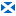2. Hyped

Messages:
1,729
Location: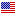You're going to need the rim diameter in millimeters (I'm nearly positive that's the units he's using), then an approximation on the profile of the tread in order to get a rough estimation of the total diameter of the rim plus the tread. If you're using stock rims, then you may just be able to google the real life size, but I don't know how accurate the game is in that regard.

Once you get the measurement of the diameter, half it to find the radius, then use that number to calculate the circumference of the tire using the formula 2 x Pi x Radius in order to obtain the final measurement.

3. GTPorsche

Messages:
21,135
There's none of that info in GT6, so good luck trying that.

KinLM likes this.
4. Hyped

Messages:
1,729
Location:You can find the rim size in GT Auto I thought. The profile is anyones guess though.

5. reefer

Messages:
752
Location:Thanks guys. Using his sheet (attached), how do others do it?

File size:
29.4 KB
Views:
21
6. Hyped

Messages:
1,729
Location:Keep everything the same except enter in the minimum and maximum for each separate gear where it tells you to, then change the engine RPM where it says, and finally change the tire circumference.

reefer likes this.
7. GTPorsche

Messages:
21,135
Stock, +1, +2 are very vague.

8. Hyped

Messages:
1,729
Location:I haven't played the game in months, so I couldn't remember. That is incredibly useless information, however.

Messages:
4,053
If you manage to find the diameter, there will be no need to half it to find the radius & then double it again. That's an unnecessary extra step.

2 x Pi x Radius
is identical to
Pi x Diameter

KinLM and CraigHyphenO like this.
10. reefer

Messages:
752
Location:Thanks guys. I can follow everything else just not the last step. Think I'd need to see a video example and follow along that way. Pity really as I would love to be able to get my gearbox bang on for each car/circuit, but as it stands it looks like that last step has beaten me.

11. Hyped

Messages:
1,729
Location:I just repeated it in the way it's been drilled into my head for so many years. Halving the diameter is a 10 second step anyways, not much time saved there.

S_Bridge likes this.
12. OdeFinn

Messages:
2,643
Location: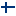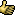Don't forget to add rubber itself.. Counting only rim isn't giving you correct speed, unless you enter too big value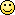13. eran0004

Messages:
8,679
Here's how to calculate the radius of the entire wheel (rubber and all).

Step one, get the following data:

• wheel speed (in km/h), let's call this S
• engine speed (in rpm), let's call this rpm
• gear ratio, let's call this G
• final drive ratio, let's call this F

(Gear ratio and final drive is in the settings tab, wheel speed and engine speed can be obtained by using the data logger feature in GT6)

Step two, calculate the total ratio and the wheel rpm:

• Total ratio (let's call this R) = F*G
• Wheel rpm (let's call this W) = rpm/R

Step three, calculate wheel radius:

• Wheel radius (in meters) = S/(W*0.377)

Example: Cobra Daytona Coupe

S = 260
rpm = 6300
G = 1.000
F = 3.090

R = 1.000 * 3.090 = 3.09
W = 6300 / 3.09 = 2038.835

Wheel radius = 260/(2038.835*0.377) = 260 / 768.64 = 0.338 meters

Edit:
I've also made a python script to automate most of this. You can copy the code below and paste in the code simulator here.

Scroll down and click on the button that says "Test your code with the code simulator", then paste the code in the "Your input" window and click "Test code". The result will be printed in the area above the input window.

Code:
kph = float(input("Speed (kph): "))
rpm = int(input("RPM: "))
g = float(input("Gear ratio: "))
fd = float(input("Final drive: "))

rpm = rpm/(g*fd)

r = kph/(rpm*0.377)

r = float(str("%.3f" %r))

return r

print(r)

Last edited: Aug 19, 2015
KinLM likes this.
14. OdeFinn

Messages:
2,643
Location:I might be little tired, but, maybe divide?

15. eran0004

Messages:
8,679
Nope, they're multiplied. Later on you divide the engine rpm with the total ratio, but to get the total ratio you need to multiply gear ratio with final drive ratio.

Edit: You can write it like this as well:

W = rpm / G / F

But I prefer it this way:

W = rpm / (G * F)

Because in the first example the outcome depends on the order of calculation - it should be left to right, but you never know with computers (or people for that matter).

While in the second example there's never a question about what to calculate first, parenthesis always have priority.

Last edited: Aug 19, 2015
OdeFinn likes this.
16. OdeFinn

Messages:
2,643
Location:Hmm, something disturbs my eye, what is that 0.377 and 768.64?
Not 0.2778, what I would understand as kmh to meters per second.
Have to get some rest and look again after that, 24h day is bit long after 4 hour sleep.

It's true that it doesn't take much time or effort but extra steps are extra opportunities to make errors.